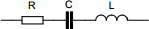# Capacitor impedance calculator

## Calculates the impedance of a capacitor at a given frequency

 Example 1: Must calculate the impedance of a 100nF capacitor at 1Mhz. The capacitor has an inductance of 0.67 nanohenries and ESR of 788 milliohms. View example
 Capacitance farads Inductance henrys ESR ohms Frequency hertzYou may use one of the following SI prefix after a value: p=pico, n=nano, u=micro, m=milli, k=kilo, M=mega, G=giga

 Results Impedance ~1.772171 ohms